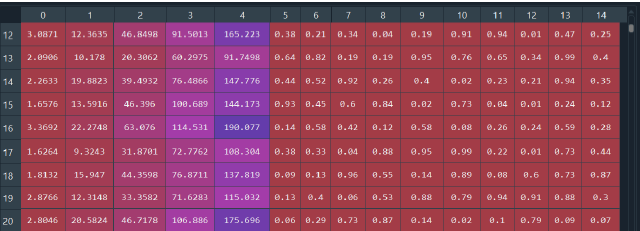# NN Models for Multi-Output Regression

I am try to build NN to solve the problem of detecting the faults. The goal is therefore to design a network which will be able to define the percentage of fault in each of the 10 points in terms of frequencies. My data have 3 feature (frequencies) and 10 prediction faults (whole value of faults are probabilities between 0 and 1). I chose using auto-encoder and MLP for deal with this problem. On the first time, I am building an MLP for multi-outputs regression, i got the figure loss on data training and data validation very small but when i teste model with on data test, i see that the percentage of fault is very different ( for example, the value correct 0.75 but my prediction value is just 0.552 ). I don’t know where are my error in my code. For auto-encoder, I just use decoder, so I take code layer correspond to 3 frequencies and output class (10 faults). i don’t think that it is better than MLP for regression
Thank you very for your help

this is my data before normalization by data[:,:5] = normalize(data[:,:5], axis=0)